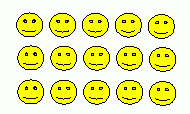Email us to get an instant 20% discount on highly effective K-12 Math & English kwizNET Programs!

#### Online Quiz (WorksheetABCD)

Questions Per Quiz = 2 4 6 8 10

### Grade 3 - Mathematics1.4 Multiplication Pictures

 Method: Count the pictures in each row. Multiply the number of patterns in each row by the total number of rows. Example: What is the multiplication form shown in the figure?This is made of 3 rows of 5 in each, we hence write as 5*3. Answer : 3 * 5 = 15 Directions: Write the multiplication form of the following figures. Also write atleast 5 examples of your own.
 Q 1: What is the multiplication form shown in the figure?3 x 2 = 84 x 2 = 84 x 2 = 9 Question 2: This question is available to subscribers only!